# IB经济学通关秘籍，这些经典例题你会了吗？

IB经济考试形式以写作类题目为主，还有简答题。

Linear demand and supply functions

Demand

Qd=a-bP

Qd: quantity demanded, and is the dependent variable

P: price, independent variable

a: the Q-intercept (or the horizontal intercept)

-b: the slope, calculated as ΔQd/ΔP.

1. Example: Qd=14-2P

2. Changes in the parameter a and shifts of the demand curve

3. Changes in parameter -b and the steepness of the demand curve

Supply

Qs=c+dP

Qs: quantity supplied, and is the dependent variable

P: price, independent variable

c: the Q-intercept (or the horizontal intercept)

d: the slope calculated as ΔQs/ΔP.

1. Example: Qs=2+2P

2. Changes in the parameter a and shifts of the supply curve

3. Changes in parameter d and the steepness of the supply curve

Using linear equations to calculate and illustrate market equilibrium

Qd = Qs (at market equilibrium)

Using the third equation, we can eliminate Qs and Qd, and solve for P:

14 – 2P = 2 + 2P ⇒ 12 = 4P ⇒ P = \$3

Substituting P into the demand equation, we have:

Qd = 14 – 2(3) ⇒ Qd = 14 – 6 = 8 units

Therefore, the equilibrium price is \$3 and the equilibrium quantity is 8,

01例题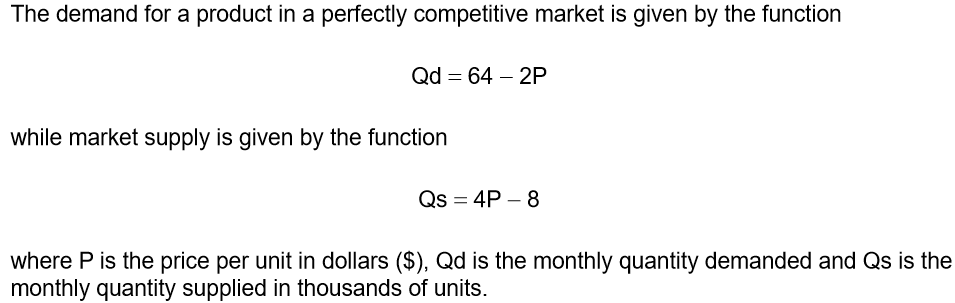(a) Outline the reason why the coefficient of price in the supply function above is positive.

(b) Calculate the equilibrium price and the equilibrium quantity in this market.

(c) Using the functions, plot the following in the grid below:

(i) a fully labelled market demand curve.

(ii) a fully labelled market supply curve.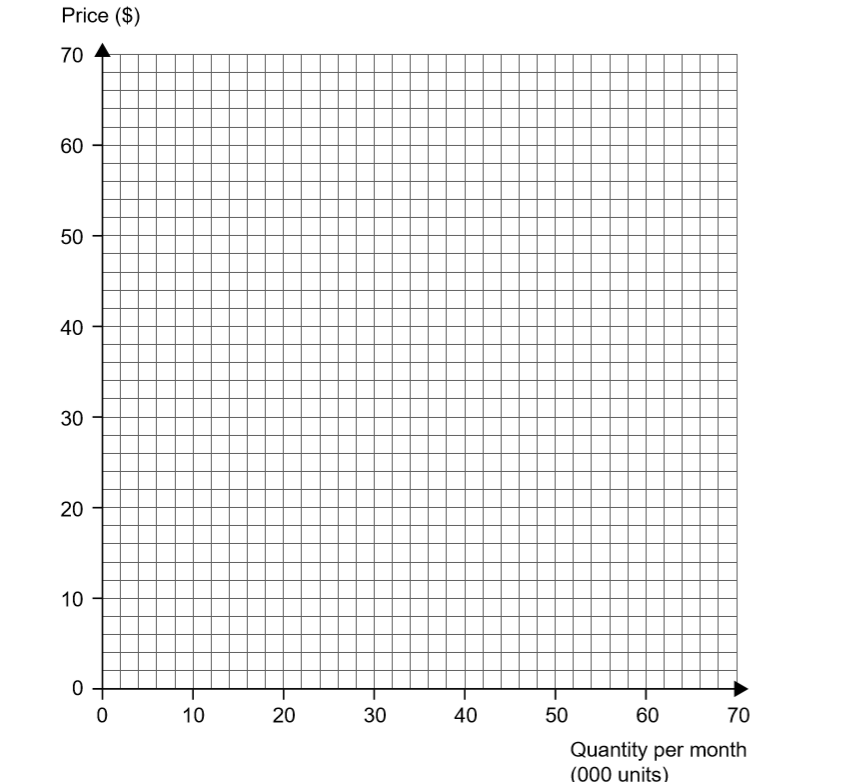02答案及详解

(a) Outline the reason why the coefficient of price in the supply function above is positive.

At higher prices, quantities supplied are greater since the profit margins of firms are higher (or reference to the idea that marginal costs are likely to increase as output increases or another valid incentive such as to gain more revenue)

(b) Calculate the equilibrium price and the equilibrium quantity in this market.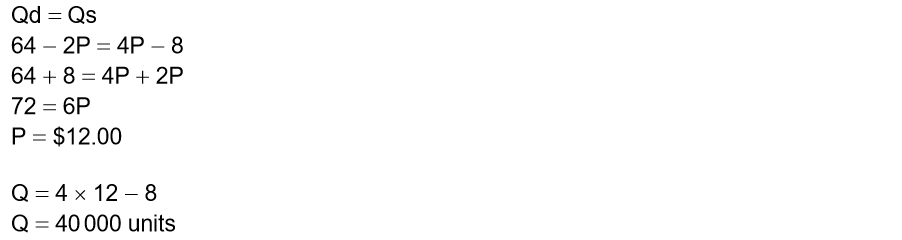(c) Using the functions, plot the following in the grid below: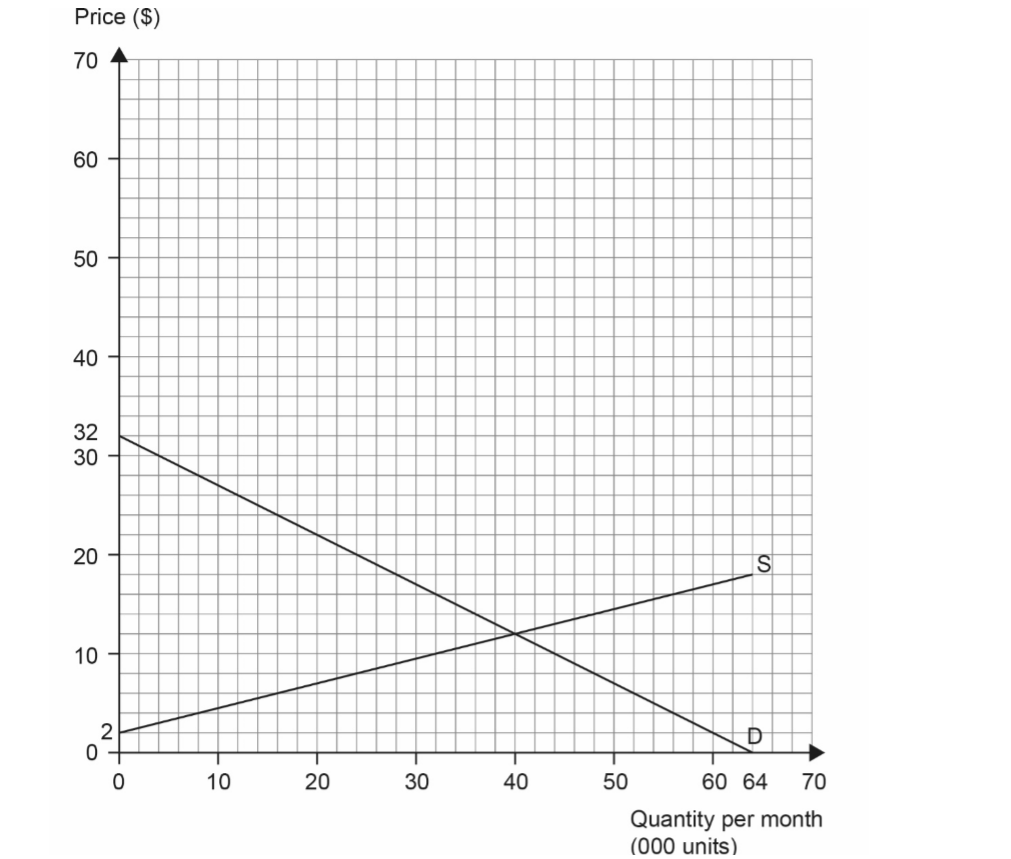03真题演练

The following diagram illustrates the weekly demand for sunglasses in the town of Picton.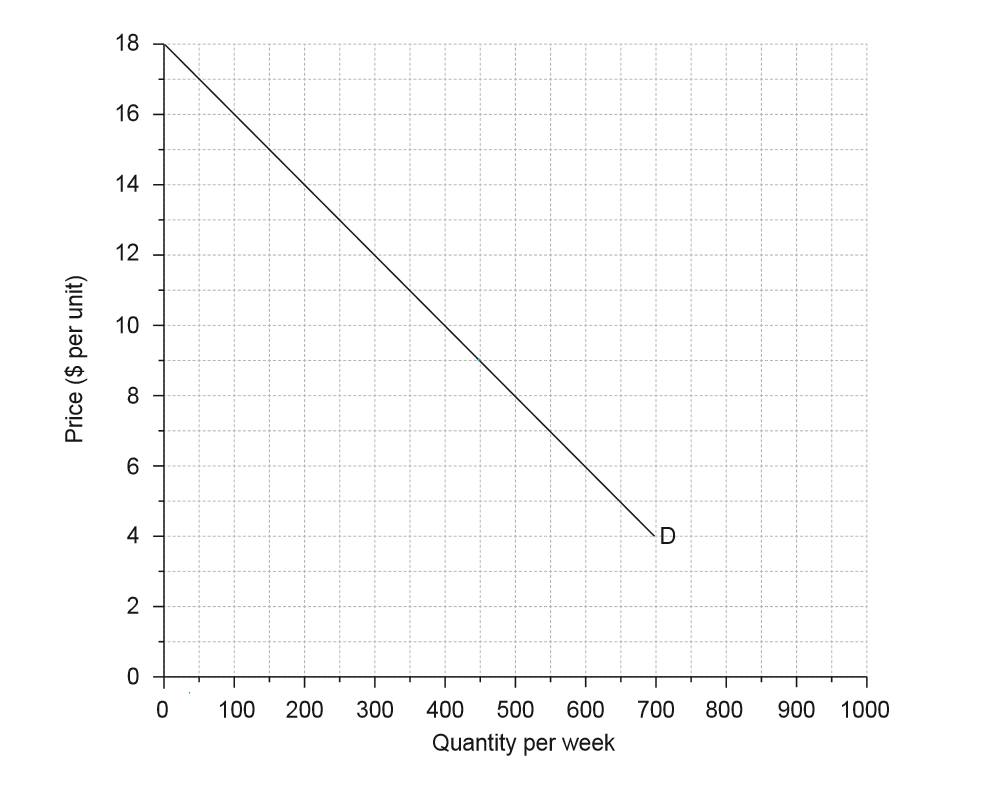The market supply and demand for sunglasses in Picton is given by the equation

Qs = – 450 + 100P

Qd = 900 - 50P

where Qs is the quantity supplied per week, Qd is the quantity demanded per week and P is

(i) On the diagram, plot and label the supply curve for sunglasses in Picton.

(ii) Calculate the equilibrium price and quantity.

• ib考试
• ib资料
• IB
• IB课程热门内容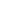在 线 客 服